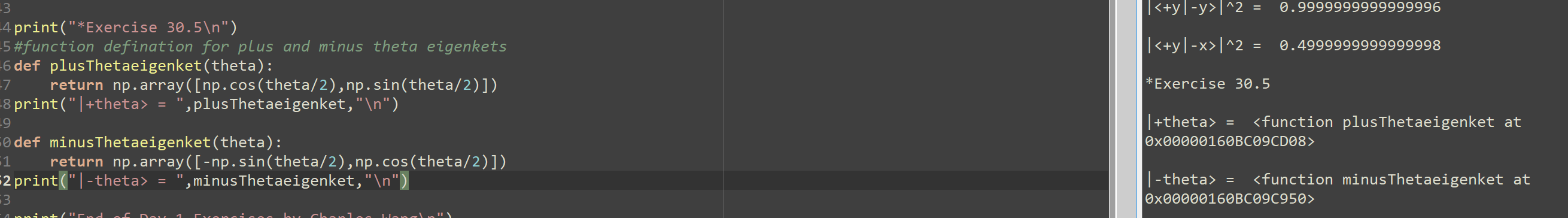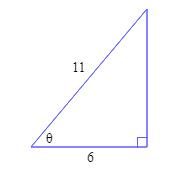# Solve each equation on the interval: 0 is less than and equal to theta is less than and equal to 360 -- 2sin2x = 1 -- --- My Answers: Let y represent 2x 2siny = 1 2siny/2 = 1/2 siny = 1/2 y = sin^-1(1/2) y = 30 2x = 30 2x/2 = 30/2 x = 15 (Quadrant 1 related homework questions

• #### Solve each equation on the interval: 0 is less than and equal to theta is less than and equal to 360 -- 2sin2x = 1 -- --- My Answers: Let y represent 2x 2siny = 1 2siny/2 = 1/2 siny = 1/2 y = sin^-1(1/2) y = 30 2x = 30 2x/2 = 30/2 x = 15 (Quadrant 1

Solve each equation on the interval:0 is less than and equal to theta is less than and equal to 360--2sin2x = 1-----My Answers:Let y represent 2x2siny = 12siny/2 = 1/2siny = 1/2y = sin^-1(1/2)y = 302x = 302x/2 = 30/2x = 15 (Quadrant 1)Quadrant 2180 - theta= 180 - 30= 1502x = 1502x/2 = 150/2x = 75Therefore, x = 15...

• #### It’s review question, I need this as soon as possible. Thank you 3) For thè diferential equation: (a) The point zo =-1 is an ordinary point. Compute the recursion formula for the coefficients of...It’s review question, I need this as soon as possible. Thank you 3) For thè diferential equation: (a) The point zo =-1 is an ordinary point. Compute the recursion formula for the coefficients of the power series solution centered at zo- -1 and use it to compute the first three nonzero terms of the power series when -1)-s and v(-1)-0....

• #### Does 'using the unit circle' just mean that I use these: In Quadrant I, theta=beta In Quadrant II, theta=180-beta In Quadrant III, theta=180+beta In Quadrant IV, theta=360-beta Because the whole lesson we did was about that sort of thing, which is all r

Does 'using the unit circle' just mean that I use these:In Quadrant I, theta=betaIn Quadrant II, theta=180-betaIn Quadrant III, theta=180+betaIn Quadrant IV, theta=360-betaBecause the whole lesson we did was about that sort of thing, which is all review from last year, but then at the end we did a quick example saying something about unit circles and every question in...

• #### Pove that 1+sin(theta)divided by 1-Sin(theta)- 1-Sin(theta) Divided by 1+Sin(theta)= 4Tan(theta)times Sec(theta) Show step by step how to change the left side of the equation to equal the right side of the equation

Pove that 1+sin(theta)divided by 1-Sin(theta)- 1-Sin(theta) Divided by 1+Sin(theta)= 4Tan(theta)times Sec(theta) Show step by step how to change the left side of the equation to equal the right side of the equation.

• #### Dont copié formé thé book oh ya dont copié formé thé book cause you Oiil inde up being triste soi remembré not toi copié frome thé book oh ya

Dont copié formé thé book oh ya dont copié formé thé book cause you Oiil inde up being triste soi remembré not toi copié frome thé book oh ya!translation in english please!

• #### def plusThetaeigenket(theta): return np.array([np.cos(theta/2),np.sin(theta/2)]) print("|+theta> = ",plusThetaeigenket,"\n") def minusThetaeigenket(theta): return np.array([-np.sin(theta/2),np.cos(theta/2)]) print("|-theta> = ",minusThetaeigenket,"\def plusThetaeigenket(theta): return np.array([np.cos(theta/2),np.sin(theta/2)]) print("|+theta> = ",plusThetaeigenket,"\n") def minusThetaeigenket(theta): return np.array([-np.sin(theta/2),np.cos(theta/2)]) print("|-theta> = ",minusThetaeigenket,"\n") Can you please help to solve where this python code went wrong for display the correct eigen ket value for theta? Thank you |<+y|-y>l^2 = 0.9999999999999996 ||<+y|-x>l^2 = 0.4999999999999998 -4 print("*Exercise 30.5\n"), -5 #function defination for plus and minus theta eigenkets -6 def plusThetaeigenket(theta): +7 return...

• #### Prove that Cot(theta)/1-Tan(theta) + Tan(theta)/1-Cot(theta) = 1+Tan(theta)+Cot(theta) must show step by step I have tried a couple of steps by changing Cot to 1-1/Cot(theta) and 1-Tan(theta) 1-1/Cot (theta)

Prove that Cot(theta)/1-Tan(theta) + Tan(theta)/1-Cot(theta) = 1+Tan(theta)+Cot(theta) must show step by step I have tried a couple of steps by changing Cot to 1-1/Cot(theta) and 1-Tan(theta) 1-1/Cot (theta)

• #### sin^2 theta + 2 cos theta -1/ (sin^2 theta +3 cos theta-3= cos^2 theta+cos theta/ (-sin^2 theta) Prove the identity

sin^2 theta + 2 cos theta -1/ (sin^2 theta +3 cos theta-3= cos^2 theta+cos theta/ (-sin^2 theta)Prove the identity.

• #### Is sin theta minus cos theta = root 2 Sin Theta then prove that sin theta + cos theta is equals to root sin theta?

If #sintheta-costheta=sqrt(2)sintheta#, then prove that, #sintheta+costheta=-sqrt(2)costheta#

• #### if cos 2 theta = -(1/3) and theta is in Quadrant 2, find sin theta, cos theta, tan theta, and draw triangle theta

if cos 2 theta = -(1/3) and theta is in Quadrant 2, find sin theta, cos theta, tan theta, and draw triangle theta

• #### Prove that Sin(theta)times Cos(theta) divided by Cos^2(theta)-Sin^2(theta)=Tan(theta) divided by 1-Tan(theta) Show step by step how to change the left to equal the right side of equasion

Prove that Sin(theta)times Cos(theta) divided by Cos^2(theta)-Sin^2(theta)=Tan(theta) divided by 1-Tan(theta) Show step by step how to change the left to equal the right side of equasion

• #### Solve each equation for o is less than and/or equal to theta is less than and/or equal to 360 -- sin^2x = 1 = cos^2x -- Work: cos^2x - cos^2x = 0 0 = 0 -- Textbook Answers: 90 and 270 -- Btw, how would you isolate for cos^2x = 0

Solve each equation for o is less than and/or equal to theta is less than and/or equal to 360--sin^2x = 1 = cos^2x--Work:cos^2x - cos^2x = 00 = 0--Textbook Answers:90 and 270--Btw, how would you isolate for cos^2x = 0? Would it be...x = cos^-1 square root of _____ ?

• #### Let sin alpha = 5/13 where 90° is less than or equal to alpha is less than or equal to 180° and cos beta = -24/25 where 180° is less than or equal to beta is less than or equal to 270° Find cos (alpha - beta) and tan (alpha + beta)

Let sin alpha = 5/13 where 90° is less than or equal to alpha is less than or equal to 180° and cos beta = -24/25 where 180° is less than or equal to beta is less than or equal to 270° Find cos (alpha - beta) and tan (alpha + beta)

• #### Given the right triangle below, find #cos theta#, #sin theta#, #tan theta#, #sec theta#, #csc theta#, and #cot theta#. Do not approximate, find exact answers and show all work?• #### Graph this: y greater than or equal to 2x-3 2x-2y is less than or equal to 1 2x-2y is less than or equal to 1 2x is less than or equal 2y x is less than or equal y how do u graph this

Graph this:y greater than or equal to 2x-32x-2y is less than or equal to 12x-2y is less than or equal to 12x is less than or equal 2yx is less than or equal yhow do u graph this? how to graph x is less than or equal to y?

• #### Prove that Cot(theta) divided by (1-Tan (theta) )+ Tan(theta) divided by (1-Cot(theta) )is equal to 1+Tan(Theta)+Cot (Theta) Show step by step how left side is equal to right side of equasion

Prove that Cot(theta) divided by (1-Tan (theta) )+ Tan(theta) divided by (1-Cot(theta) )is equal to 1+Tan(Theta)+Cot (Theta) Show step by step how left side is equal to right side of equasion

• #### 1)Use the definitions sin0(theta) = y/r, cos0(theta) = x/r and/or tan0(theta) = y/x to prove that cot0(theta) = 1/tan0(theta) 2)For all 0(theta) prove: a)cos(t) = 1/sec0(theta) b

1)Use the definitions sin0(theta) = y/r, cos0(theta) = x/r and/or tan0(theta) = y/x to prove that cot0(theta) = 1/tan0(theta) 2)For all 0(theta) prove: a)cos(t) = 1/sec0(theta) b. sec(t) = 1/cos0(theta)

Free Homework App